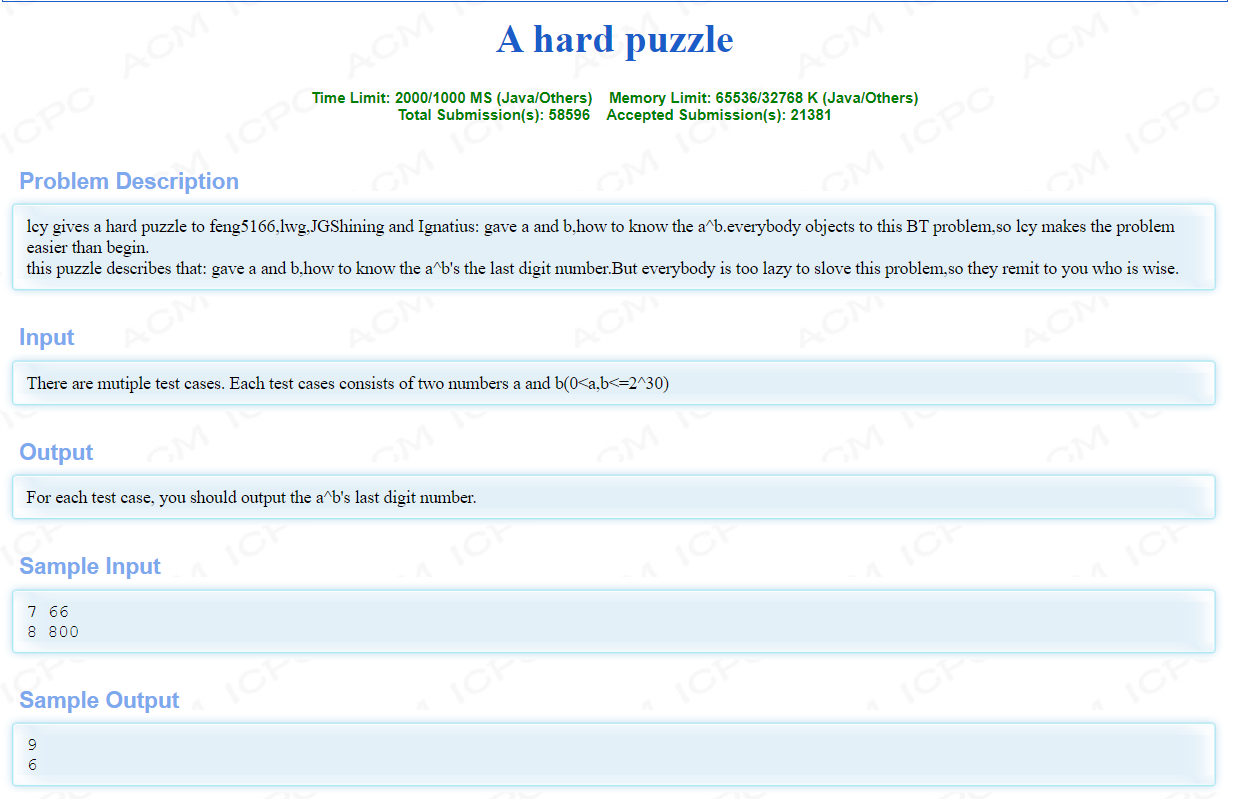POJ1097

# 题面：# 翻译：

lcy给了feng5166,lwg,JGShining和Ignatius一个难题:给了a和b，如何知道a^b。每个人都反对这个BT问题，所以lcy使这个问题比开始容易。

7 66
8 800

9
6

# 思路：

1:当b为偶数时，a^b = = a^(b/2) * a^(b/2)
2:当b为奇数时，a^b = =a * (a^(b/2)) * (a^(b/2))

int quick_mi(int a,int b)
{
int ans=1;
while(b)
{
if(b&1)//奇数隔单,位运算更快，其实还可以写为，b%2==1
{
ans=ans*a;
}
a=a*a;//偶数折半
b=b>>1;//位运算折半，其实还可以写为b=b/2
}
return ans;
}


1:根据数学公式:a * b%m=(a%m)*(b%m)%m

int quick_mi(int a,int b,int c)
{
int ans=1;
while(b)
{
if(b&1)//奇数隔单
{
ans=ans*a%c;
}
a=a*a%c;//偶数折半
b=b>>1;
}
return ans;
}



# 参考代码：

#include<iostream>
using namespace std;
int main()
{
long long a,b,ans,i;
while(cin>>a>>b)
{
long long ans=1;
while(b)
{
if(b&1)
{
ans=(ans%10*a)%10;
}
a=(a%10*a%10)%10;
b=b>>1;
}
cout<<ans<<endl;
}
}


©️2019 CSDN 皮肤主题: 数字20 设计师: CSDN官方博客